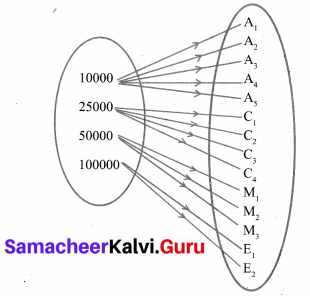Samacheer Kalvi 10th Maths Solutions Chapter 1 Relations and Functions Ex 1.2

Tamilnadu Samacheer Kalvi 10th Maths Solutions Chapter 1 Relations and Functions Ex 1.2

10th Maths Exercise 1.2 Samacheer Kalvi Question 1.
Let A = {1, 2, 3, 7} and B = {3, 0, -1, 7}, which of the following are relation from A to B ?
(i) R1 = {(2, 1), (7, 1)}
(ii) R2 = {(-1, 1)}
(iii) R3 = {(2, -1), (7, 7), (1, 3)}
(iv) R4 = {(7,-1), (0, 3), (3, 3), (0, 7)}
(i) A = {1, 2, 3, 7}, B = {3, 0,-1, 7}
Solution:
R1 = {(2,1), (7,1)}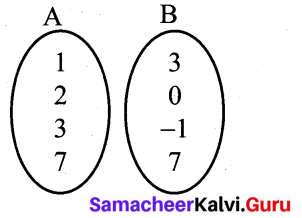It is not a relation there is no element as 1 in B.
(ii) R2 = {(-1, 1)}
It is not [∵ -1 ∉ A, 1 ∉ B]
(iii) R3 = {(2, -1), (7, 7), (1, 3)}
It is a relation.
R4 = {(7,-1), (0, 3), (3, 3), (0, 7)}
It is also not a relation. [∵ 0 ∉ A]

Ex 1.2 Class 10 Samacheer Question 2.
Let A = {1, 2, 3, 4, ….., 45} and R be the relation defined as “is square of ” on A. Write R as a subset of A × A. Also, find the domain and range of R.
A = {1,2, 3, 4 . . . . 45}
The relation is defined as “is square of’
R = {(1,1) (2, 4) (3, 9)
(4, 16) (5,25) (6, 36)}
Domain of R = {1, 2, 3, 4, 5, 6}
Range of R = {1, 4, 9, 16, 25, 36}

10th Maths Exercise 1.2 Answers Samacheer Kalvi Question 3.
A Relation R is given by the set {(x, y) /y = x + 3, x ∈ {0, 1, 2, 3, 4, 5}}. Determine its domain and range.
Solution:
x = {0, 1, 2, 3, 4, 5}
y = x + 3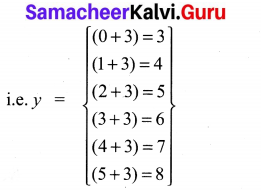⇒ y = {3, 4, 5, 6, 7, 8}
R = {(x, y)}
= {(0, 3),(1, 4),(2, 5),(3, 6), (4, 7), (5, 8)}
Domain of R = {0, 1, 2, 3, 4, 5}
Range of R = {3, 4, 5, 6, 7, 8}

10th Maths Exercise 1.2 In Tamil Samacheer Kalvi Question 4.
Represent each of the given relation by (a) an arrow diagram, (b) a graph and (c) a set in roster form, wherever possible.
(i) {(x, y)|x = 2y,x ∈ {2, 3, 4, 5},y ∈ {1, 2, 3, 4)
(ii) {(x, y)|y = x + 3, x, y are natural numbers <10}
Solution:
(i){(x, y)|x = 2y, x ∈ {2, 3, 4, 5},y ∈ {1, 2, 3, 4}} R = (x = 2y)
2 = 2 × 1 = 2
4 = 2 × 2 = 4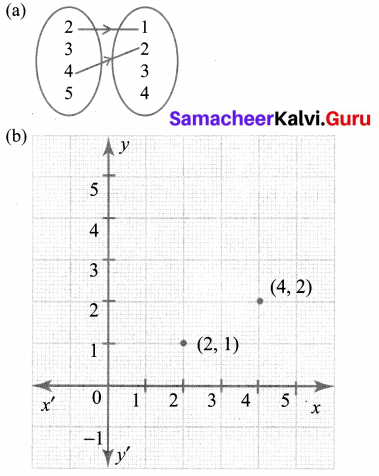(c) {(2, 1), (4, 2)}
(ii) {(x, y)|y = x + 3, x,+ are natural numbers <10}
x = {1, 2, 3, 4, 5, 6, 7, 8, 9} R = (y = x + 3)
y = {1, 2, 3, 4, 5, 6, 7, 8, 9}
R = {(1, 4), (2, 5), (3, 6), (4, 7), (5, 8), (6, 9)}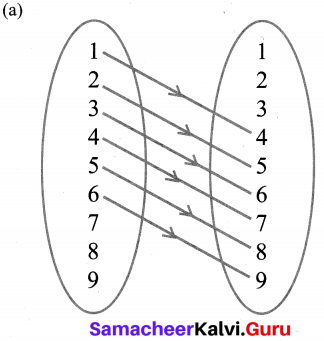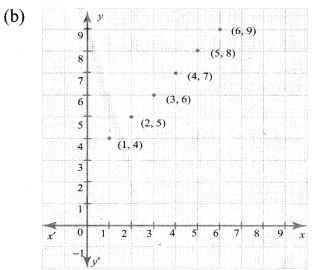(c) R = {(1, 4), (2, 5), (3, 6), (4, 7), (5, 8), (6, 9)}

10th Maths Exercise 1.2 Samacheer Kalvi Question 5.
A company has four categories of employees given by Assistants (A), Clerks (C), Managers (M) and an Executive Officer (E). The company provide ₹10,000, ₹25,000, ₹50,000 and ₹1,00,000 as salaries to the people who work in the categories A, C, M and E respectively. If A1, A2, A3, A4 and As were Assistants; C1, C2, C3, C4 were Clerks; M1, M2, M3 were managers and E1,E2 were Executive officers and if the relation R is defined by xRy, where x is the salary given to person y, express the relation R through an ordered pair and an arrow diagram.
Solution:
A – Assistants → A1, A2, A3, A4, A5
C – Clerks → C1, C2, C3, C4
D – Managers → M1, M2, M3
E – Executive officer → E1, E2
(a) R = {(10,000, A1), (10,000, A2), (10,000, A3),
(10,000, A4), (10,000, A5), (25,000, C1),
(25,000, C2), (25,000, C3), (25,000, C4),
(50,000, M1), (50,000, M2), (50,000, M3),
(1,00,000, E1), (1,00,000, E2)}

(b)